# Barrel 3

Barrel with water weights 118 kg. When we get off 75% of water, it weights 35 kg. How many kg has an empty barrel?

x =  7.33 kg

### Step-by-step explanation:

x+y=118
x+(1-75/100)y=35

x+y=118
x+(1-75/100)•y=35

x+y = 118
100x+25y = 3500

x = 22/3 ≈ 7.333333
y = 332/3 ≈ 110.666667

Our linear equations calculator calculates it.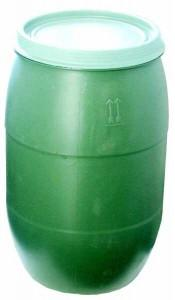Did you find an error or inaccuracy? Feel free to write us. Thank you!Tips to related online calculators
Do you have a linear equation or system of equations and looking for its solution? Or do you have a quadratic equation?
Tip: Our volume units converter will help you with the conversion of volume units.

## Related math problems and questions:

• Water containerContainer with water weighs 1.48 kg. When we cast 75% of water container of water weight 0.73 kg. How heavy is an empty container?
• Barrel of oilBarrel of oil weighs 283 kg. When it mold 26% oil, weighed 216 kg. What is the mass of the empty barrel?
• The water barrel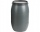The water barrel weighs 122 kg. If we pour 75% of the water out of it, it will weigh 35 kg. What is the weight of the barrel?
• Barrel 4Barrel of water weighs 63 kg. After off 75% water, the weight of the barrel with water is 21 kg. How many kg weigh empty barrel and how many kgs water in it?
• Barrel 2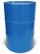Empty barrel weighs 7 kg. When it filled with water up to 45% height, weighs 60 kg. How heavy is barrel full of water?
• Barrel with liquid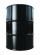Barrel with grain weight 297 kg. When it shed 48% of grain, ha weight 174 kg. What is the weight of an empty barrel?
• Container with water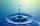The weight of a container with water is 2.48 kg. If the cast 75% water, the container with water has a weight of 0.98 kg. Determine the weight of the empty container. How much water was originally in the container?
• The barrel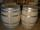The barrel is 135 cm high. If we fill it to a height of 60 cm and it weighs 163 kg. If we fill it to a height of 80 cm, it weighs 213 kg. How many kg weighs a full and how much empty barrel?
• Barrel with water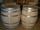The water barrel has a weight of 52 kg. When we pour a quarter of the water, the weight of the barrel is reduced to 40 kg. Determine the weight of the empty barrel.Number 118 divide into two addends, so the first addend is 69 greater than 75% of the second addend.
• Barrel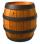The barrel is filled with water up to 57% of its capacity. When we add two buckets will be filled to 73% of its capacity. What is the volume of a barrel, if the volume of the bucket is 12 liters?
• Nectar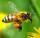Nectar collected by bees contains 70% water. From the nectar of the some process produces honey which contains 19% water. How many kg of nectar bees need to collect to make 1 kg of honey?
• Camel and water84% of the camel's weight is water. After drinking, its weight increased to 832 kg, and water accounted for 85% of its weight. How much did it weigh before drinking?
• Rain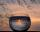How many mm of water rained the roof space 75 m2 if the empty barrel with a radius of 8 dm and height 1.2 m filled to 75% its capacity? :-)
• Reservoir + waterThe reservoir filled with water weighs 12 kg and after pouring off three-quarters of the water weights 3 kg. Calculate the weight and volume of the reservoir.
• Wood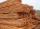Wood contains 12% water. 1 m3 of wood weighs 650 kg. How many liters of water it contains?
• Acid evaporationHow many kilograms of water do we have to evaporate from 100 kg of 32% acid to make it 80% concentration?